Question

For each problem, find the volume of the solid that results when the region enclosed by...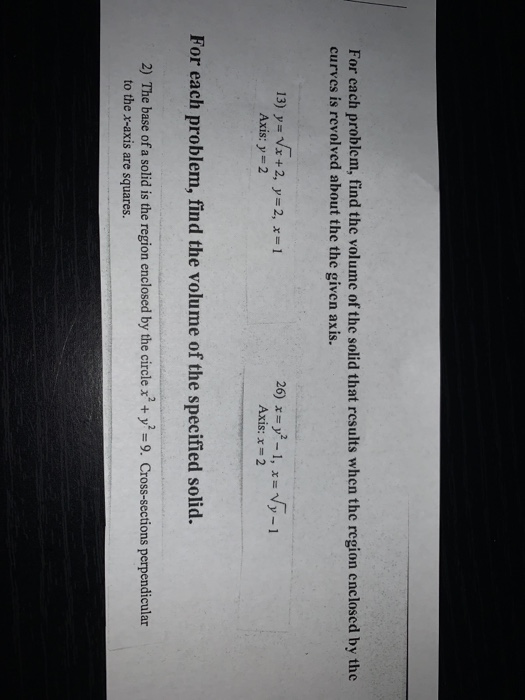For each problem, find the volume of the solid that results when the region enclosed by the curves is revolved about the given axis.
For each problem, find the volume of the solid that results when the region enclosed by the curves is revolved about the the given axis. 13) y= Vx+2, y=2, x=1 Axis: y = 2 26) *= y2 - 1, x= Vy-1 Axis: x = 2 For each problem, find the volume of the specified solid. 2) The base of a solid is the region enclosed by the circle x' + y = 9. Cross-sections perpendicular to the x-axis are squares.

We need at least 10 more requests to produce the answer.

0 / 10 have requested this problem solution

The more requests, the faster the answer.

All students who have requested the answer will be notified once they are available.

Earn Coins

Coins can be redeemed for fabulous gifts.

Similar Homework Help Questions
• For each problem, find the volume of the solid that results when the region enclosed by...For each problem, find the volume of the solid that results when the region enclosed by the curves is revolved about the the x-axis. You may use the provided graph to sketch the curves and shade the enclosed region 1) у-х?+1 2) x4 1 y=1 x= 2 у 32 A y 6 6 4 -6 -2 4 For each problem, find the volume of the solid that results when the region enclosed by the curves is revolved about the the...

• 1. Find the volume of the solid that results when the region enclosed by y =...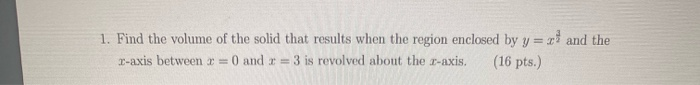1. Find the volume of the solid that results when the region enclosed by y = zand the z-axis between x = 0 and x = 3 is revolved about the c-axis. (16 pts.)

• Find the area of the region enclosed by the curves: x = -sec^2 y, x = sec^2 y, y = 0, y = pi/4 Using the method of cylindrical shells to find the volume of the solid that results when the region enclo...

Find the area of the region enclosed by the curves: x = -sec^2 y, x = sec^2 y, y = 0, y = pi/4 Using the method of cylindrical shells to find the volume of the solid that results when the region enclosed by the curves is revolved around the y axis. y = sqrt (x+1), y = 1, x = 1 y = 3 sqrt x, y =0, x =1 Find the volume of the solid that results when...

• 3 1. Find the volume of the solid that results when the region enclosed by y...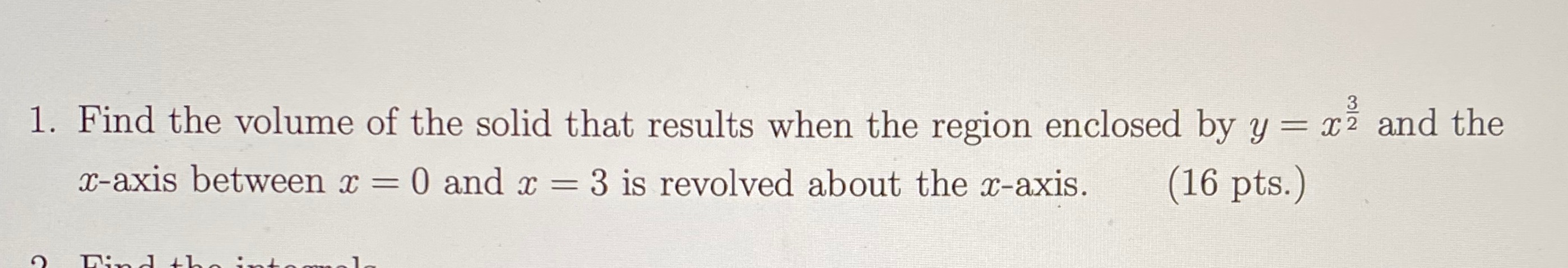3 1. Find the volume of the solid that results when the region enclosed by y = xż and the x-axis between x = 0 and x = 3 is revolved about the x-axis. (16 pts.) Dinh

• 2. (14 points) Find the volume of the solid that results when the region enclosed by...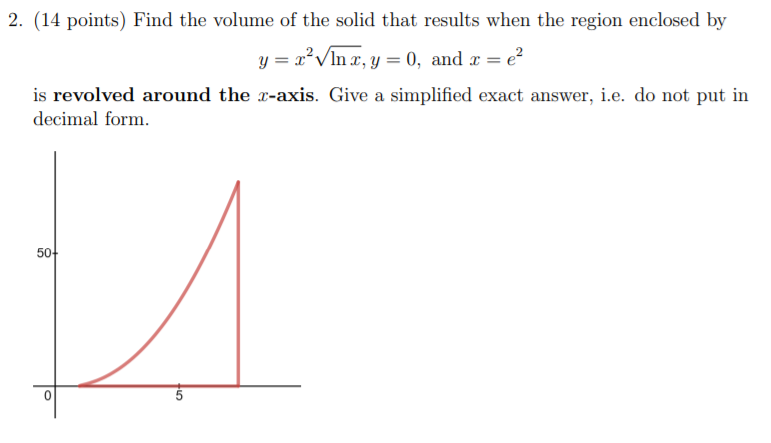2. (14 points) Find the volume of the solid that results when the region enclosed by y= x² VIn x, y = 0, and x = €2 is revolved around the r-axis. Give a simplified exact answer, i.e. do not put in decimal form. 50+

• Find the volume of the solid that results when the region enclosed by y= 2x –...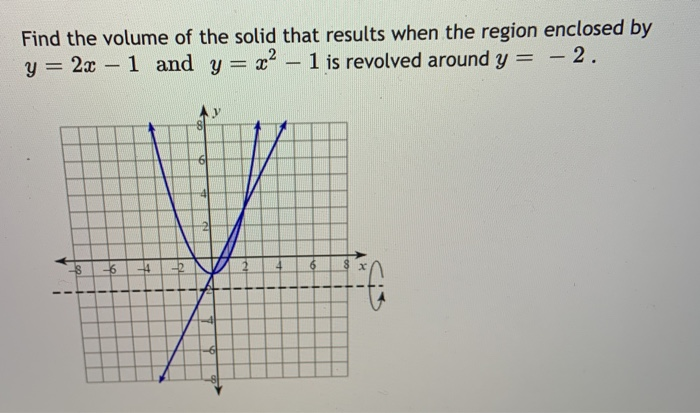Find the volume of the solid that results when the region enclosed by y= 2x – 1 and y= 22 – 1 is revolved around y = – 2. 41 XV S -6 7 1

• Find the volume of the solid generated by revolving the region R bounded by the graphs...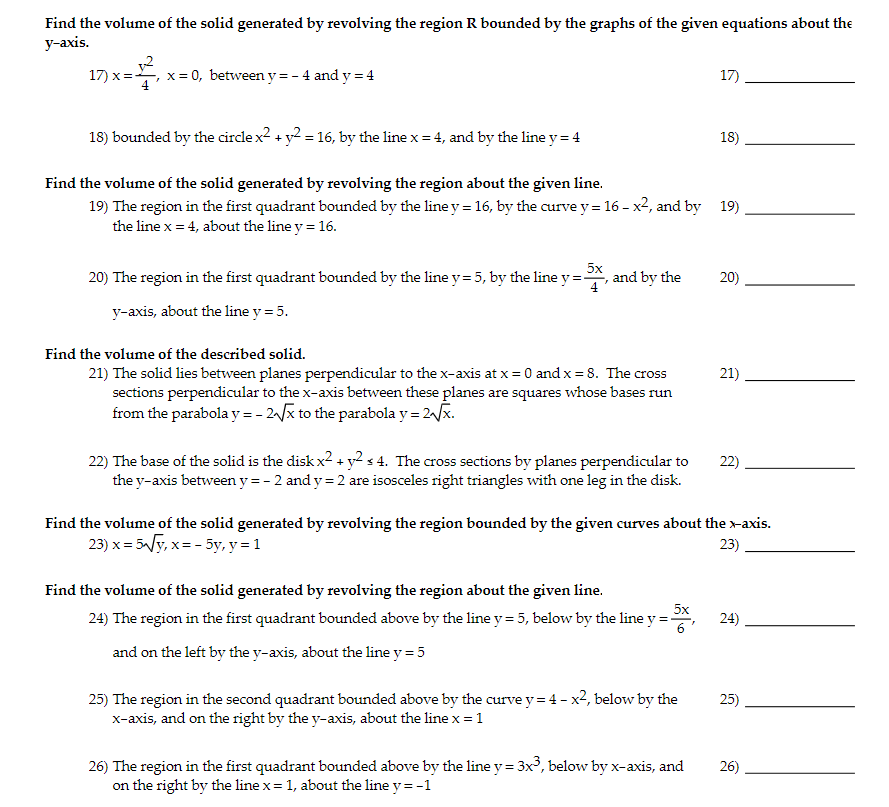Find the volume of the solid generated by revolving the region R bounded by the graphs of the given equations about the y-axis. 17)x= x=0, between y=- 4 and y = 4 17) 18) bounded by the circle x2 + y2 = 16, by the line x = 4, and by the line y = 4 18) Find the volume of the solid generated by revolving the region about the given line. 19) The region in the first quadrant bounded...

• Question 1 (2 points) ✓ Saved The base of a solid, s, is the region enclosed...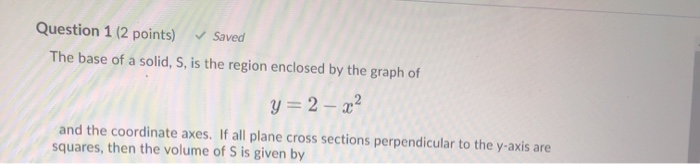Question 1 (2 points) ✓ Saved The base of a solid, s, is the region enclosed by the graph of y = 2 - 22 and the coordinate axes. If all plane cross sections perpendicular to the y-axis are squares, then the volume of S is given by Question 2 (2 points) The region enclosed by the graph of y = 1 and y=sin(x) from X = 0 to x = is rotated about about the x-axis. What is the...

• Problem 2 (1) Find the area enclosed by the curves y 2 and y-4z-z2 (2) Find the volume of the solid whose base is the triangular region with vertices(0, 0), (2, 0), and (0,1). Cross-sections perpendi...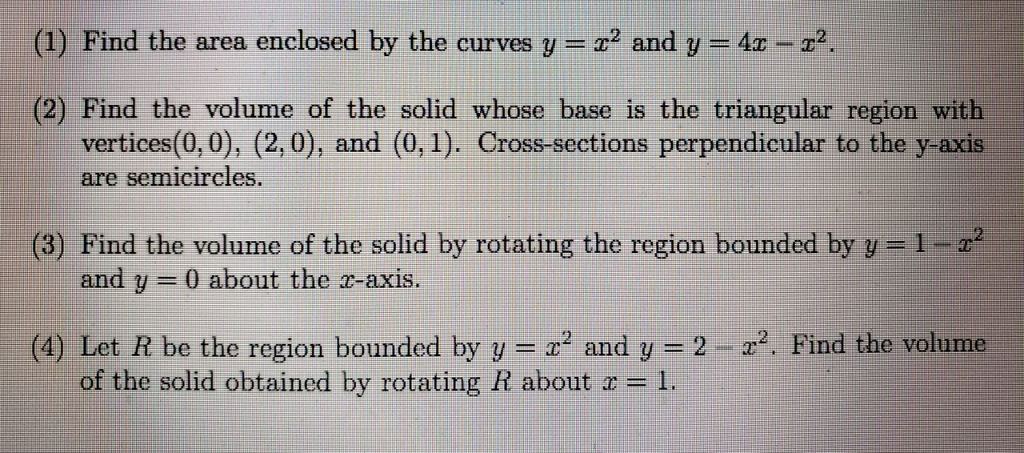Problem 2 (1) Find the area enclosed by the curves y 2 and y-4z-z2 (2) Find the volume of the solid whose base is the triangular region with vertices(0, 0), (2, 0), and (0,1). Cross-sections perpendicular to the y-axis semicircles. are (3) Find the volume of the solid by rotating the region bounded by y=1-z2 and y-0 about the r-axis. 2-z2. Find the volume (4) Let R be the region bounded by y--x2 and y of the solid obtained by...

• Find the volume V of the described solid S. The base of S is the region...

Find the volume V of the described solid S. The base of S is the region enclosed by the parabola y = 4 − 2x2 and the x−axis. Cross-sections perpendicular to the y−axis are squares.